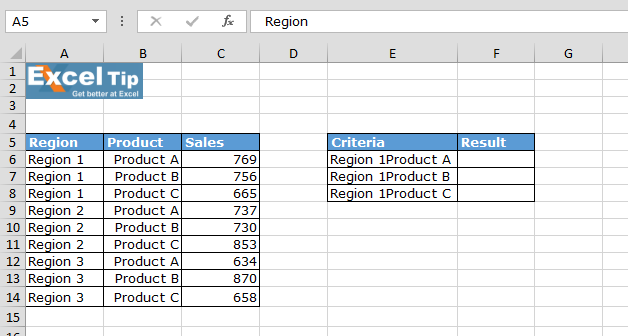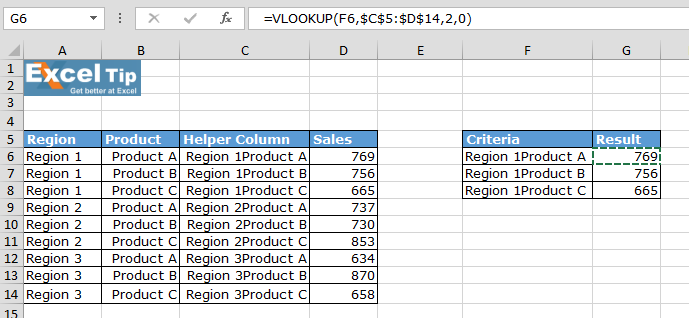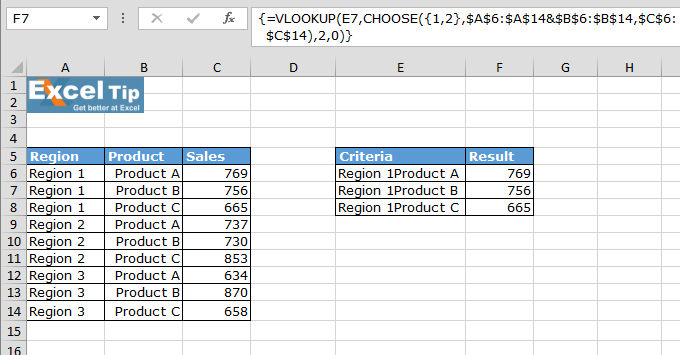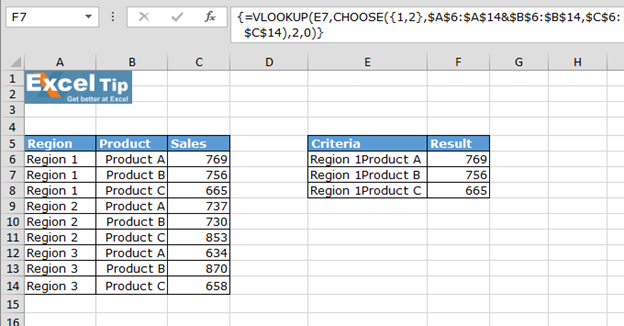# Using Choose function along with Vlookup

Consider a scenario, in which we are required to use helper column to get the output using VLOOKUP function. In this article, we will let you know how to get the output without using helper column by using the combination of VLOOKUP  &  CHOOSE functions.

Let’s take an example and understand:-
We have a sales report for 3 Region & 3 Products. In column E, there are criteria’s listed which are combination of Region & Product. We want a formula that will derive the result in column F by looking up value in column A, B & C.The common way of getting the output is to use Helper column:• In cell G2 the formula would be =VLOOKUP(F2,\$C\$2:\$D\$10,2,0)To get the output without using Helper column, use below given formula:-

• In cell F2 the formula would be: {=VLOOKUP(E2,CHOOSE({1,2},\$A\$2:\$A\$10&\$B\$2:\$B\$10,\$C\$2:\$C\$10),2,0)}

Note:- This is an array formula which requires formula to be enclosed with curly brackets by using CTRL + SHIFT + ENTER.Hence, using Vlookup & Choose functions together we need not to use Helper column to find the result.We would love to hear from you, do let us know how we can improve, complement or innovate our work and make it better for you. Write us at info@exceltip.com

## Users are saying about us...

1.the formula is not giving the proper value

Terms and Conditions of use

The applications/code on this site are distributed as is and without warranties or liability. In no event shall the owner of the copyrights, or the authors of the applications/code be liable for any loss of profit, any problems or any damage resulting from the use or evaluation of the applications/code.